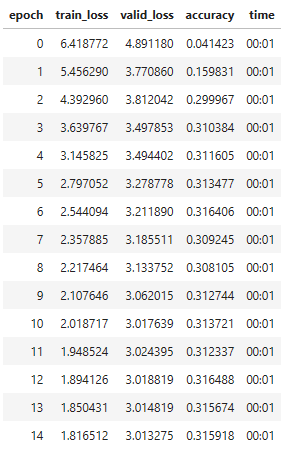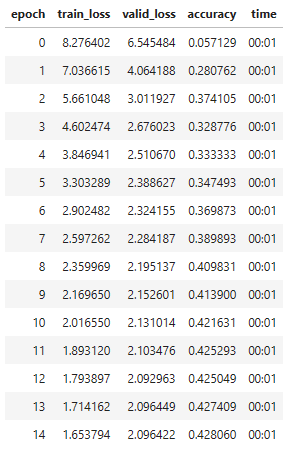# Fastbook - GRU from Scratch

In chapter 12, we are asked to create a GRU (Gated Recurrent Unit) from scratch, and to compare our results to PyTorch’s implementation.

I have done so, but the accuracy of my version is significantly less than PyTorch’s:

Mine:Pytorch:Also, the validation loss stops improving much earlier, and the accuracy does not improve. I am convinced it is a problem with my own implementation, and I don’t know where I am making the error.

Here is the code for the module:

``````class GRUCell(Module):
def __init__(self, ni, nh):
super(GRUCell, self).__init__()
self.reset_gate = nn.Linear(ni + nh, nh)
self.update_gate = nn.Linear(ni + nh, nh)
self.candidate = nn.Linear(ni + nh, nh)

def forward(self, input, hidden):
out_seq = []
#print(input.shape)
#we have an input tensor of shape n_hidden, sl, n_hidden
for in_step in input.permute(1,0,2):
#print(in_step.shape)
combined = torch.cat([in_step, hidden], dim = 1)
reset_gate = torch.sigmoid(self.reset_gate(combined))
update_gate = torch.sigmoid(self.update_gate(combined))
combined_reset = torch.cat([in_step, reset_gate * hidden], dim = 1)
candidate_hidden = torch.tanh(self.candidate(combined_reset))
new_hidden = (1 - update_gate) * hidden + update_gate * candidate_hidden
out_seq.append(new_hidden)
hidden = new_hidden.clone().detach()

#print(f'out_seq shape: {out_seq.shape}')
#we have an output tensor of shape sl, n_hidden, n_hidden
out_seq = torch.stack(out_seq, dim = 1)
return out_seq
``````

Here is a link to the full notebook on Kaggle, in case the issue exists elsewhere: GRU From the Basics

I am a beginner, so if anyone could offer any pointers, it would be much appreciated.